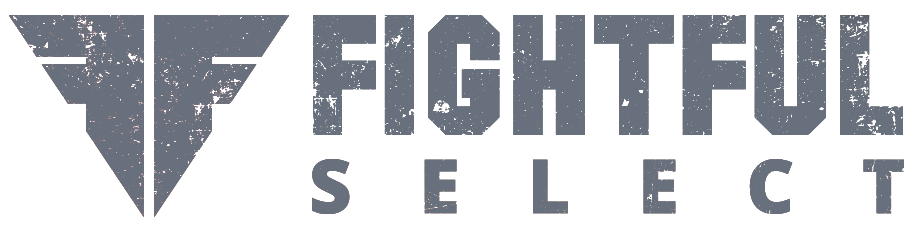# Bellator 199 Fighter Salaries

Here are the Bellator 199 fighter salaries:

Main Card

Ryan Bader (\$150,000 + no win bonus = \$150,000) def. Muhammed Lawal (\$150,000)
Jon Fitch (\$60,000 + \$60,000 = \$120,000) def. Paul Daley (\$30,000)
Aaron Pico (\$35,000 + \$35,000 = \$70,000) def. Lee Morrison (\$12,000)
Cheick Kongo (\$150,000 + no win bonus = \$150,000) def. Javy Ayala (\$30,000)
Adam Piccolotti (\$18,000 + \$18,000 = \$36,000) def. Carrington Banks (\$14,000)UFC 276 Highlights: Alexander Volkanovski Makes His Case As Pound For Pound Best

Preliminary Card

Deron Winn (\$1,200 + \$1,200 = \$2,400) def. Ahmed White (\$1,200)
Justin Tenedora (\$1,500 + \$1,500 = \$3,000) def. David Rivera (\$1,200)
Gaston Bolanos (\$5,000 + no win bonus = \$5,000) def. Malcolm Hill (\$1,200)
James Terry (\$3,000 + \$3,000 = \$6,000) def. Danasabe Mohammed (\$1,500)
Amber Leibrock (\$10,000 + \$10,000 = \$20,000) def. Janay Harding (\$10,000)
Jordan Williams (\$1,500 + \$1,500 = \$3,000) def. Brandon Hester (\$1,750)
Hyder Amil (\$1,200 + \$1,200 = \$2,400) def. Elias Anderson (\$1,200)
Ignacio Ortiz (\$1,200 + \$1,200 = \$2,400) def. Matt Aragoni (\$1,200)
Mark Climaco (\$1,200 + \$1,200 = \$2,400) def. Daniel Oseguera (\$1,200)
J.J. Okanovich (\$1,200 + \$1,200 = \$2,400) def. Hugo Lujan (\$1,200)
Thomas Ponce de Leon (\$1,200 + \$1,200 = \$2,400) def. Dominic Sumner (\$1,200)
Hohelin Hernandez (\$1,500 + \$1,500 = \$3,000) def. Josh San Diego (\$2,000)
Josh Paiva (\$2,500 + \$2,500 = \$5,000) def. Adam Antolin (\$2,500)
Cass Bell (\$1,200 + \$1,200 = \$2,400) def. Khai Wu (\$1,200)

Notes

* Ryan Bader, King Mo & Cheick Kongo tied for the highest salary at \$150,000 each

* 9 fighters tied for the lowest salary at \$1,200 each

* The biggest gap in fighter salary for a single bout is \$120,000 for the Cheick Kongo/Javy Ayala encounter